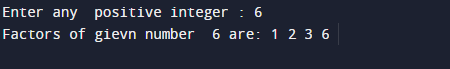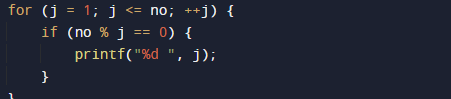# C Program to Find Factor of a Given Number

In this tutorial you will learn about the C Program to Find Factor of a Given Number and its application with practical example.

In this tutorial, we will learn to create a C program that  will find Factors of a number  using C programming.

## Prerequisites.

Before starting with this tutorial we assume that you are best aware of the following C programming topics:

• Operators.
• If else statement.
• C for Loop.
• Basic input/output.

## What Is Factor of number is?

Factors:=> So factors of a number are numbers that divide the number completely and remainder of divisor is equal to zero.

## Example:->

The number 6 has four factors: 1, 2, 3,  and 6 because all this number divide the number six completely.

## C Program to Find Factor of a Given Number.

In this program we will  find factor of given number  using if else statement and for loop. We would first declared and initialized the required variables. Next, we would prompt user to input the number . Later we will find factors.

## OutputIn this program  we will take a positive integer from user by prompting  and with the help of loop and if else statement  we will displays all the positive factors of that number.

In the above program, we have first declared and initialized a set variables required in the program.

• no = It will hols number given by user.
• j= for iteration of loop and finding  factor.

So the logic behind computing factors of a number is we will run a loop from 1 to no, increment 1  in each iteration.
The loop structure should look like thisWithin the loop  in each iteration we check the condition that j is a factor of no or not. To check factor we use divisibility of number by using  modulo division

i.e. if( no % j == 0) then j is a factor of no If j is a factor of no then we will print the value of j.So we will get the required factors of given number.

In this tutorial we have learn about the C Program to Find Factor of a Given Number and its application with practical example. I hope you will like this tutorial.